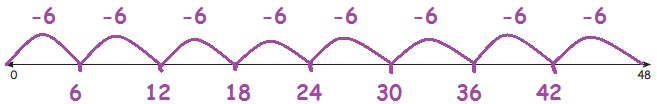Home | | Maths 3rd Std | Equal Grouping

# Equal Grouping

Division can also be done by Equal grouping.

Equal Grouping

Division can also be done by Equal grouping.

This is Rangamma’s shop.

She used to arrange the vegetables into equal groups called as kooru to sell them.1. Rangamma has 40 lemons and arranged 5 lemons in each group. Shall we find the number of groups by grouping the lemons.Show this in the given number line.The number statement can be written as 40 ÷ 5 = 8

2. Rangamma has 36 coconuts and arranges them into group of 4 each. Find the number of groups.The number line can be drawn asThe number statement is 36 ÷ 4 = 9

3. Rangamma has got 48 gooseberries and arranges them into group of 6 each. Find the number of groups.The number line can be drawn asThe number statement is 48 ÷ 6 = 8

4. Find few other ways that Rangamma can group these 48 gooseberries and write the number statements.

a. 48 ÷ 1 = 48

b. 48 ÷ 2 = 24

c. 48 ÷ 3 = 16

d. 48 ÷ 4 = 12

e. 48 ÷ 6 = 8

f. 48 ÷ 8 = 6

g. 48 ÷ 12 = 4

h. 48 ÷ 16= 3

i. 48 ÷ 24 = 2

j. 48 ÷ 48 = 1

Tags : Numbers | Term 3 Chapter 2 | 3rd Maths , 3rd Maths : Term 3 Unit 2 : Numbers
Study Material, Lecturing Notes, Assignment, Reference, Wiki description explanation, brief detail
3rd Maths : Term 3 Unit 2 : Numbers : Equal Grouping | Numbers | Term 3 Chapter 2 | 3rd Maths